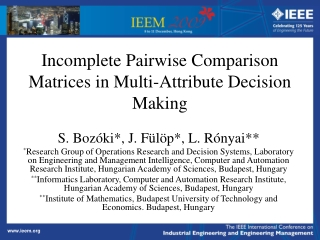DownloadDownload PresentationIncomplete Pairwise Comparison Matrices in Multi-Attribute Decision Making

# Incomplete Pairwise Comparison Matrices in Multi-Attribute Decision Making

Download Presentation## Incomplete Pairwise Comparison Matrices in Multi-Attribute Decision Making

- - - - - - - - - - - - - - - - - - - - - - - - - - - E N D - - - - - - - - - - - - - - - - - - - - - - - - - - -
##### Presentation Transcript

1. Incomplete Pairwise Comparison Matrices in Multi-Attribute Decision Making S. Bozóki*, J. Fülöp*, L. Rónyai** *Research Group of Operations Research and Decision Systems, Laboratory on Engineering and Management Intelligence, Computer and Automation Research Institute, Hungarian Academy of Sciences, Budapest, Hungary **Informatics Laboratory, Computer and Automation Research Institute, Hungarian Academy of Sciences, Budapest, Hungary **Institute of Mathematics, Budapest University of Technology and Economics. Budapest, Hungary

2. Outline • Pairwise comparison matrix • Incomplete pairwise comparison matrix • Eigenvalue minimization • Incomplete Logarithmic Least Squares • Algorithms • Example

3. Pairwise comparison matrix It is one of the most often applied technique in Multi-Attribute Decision Making for weighting the criteria and evaluation of the alternatives. Pairwise comparison matrix is a basic concept of the Analytic Hierarchy Process (AHP) developed by Thomas Saaty in 1977.

4. Pairwise comparison matrix A (complete) pairwise comparison matrix A = [aij]i,j=1,…,n is a matrix of size n×n with the properties as follows: aij > 0; aii = 1; aij = 1/aji for i,j=1,…,n, where aij is the numerical answer given by the decision maker for the question “How many times Criterion i is more important than Criterion j?” The weighting problem is to find the n-dimensional positive weight vector w = (w1,w2,…,wn)Tsuch that the appropriate ratios of the components of w reflect, or, at least, approximate all the aij values (i,j=1,…,n), given by the decision maker.

5. Incomplete pairwise comparison matrix

6. Incomplete pairwise comparison matrix • Harker (1987) • Kwiesielewicz (1996) • Shiraishi, Obata, Daigo (1997, 1998, 2002) • van Uden (2002)

7. Undirected graph representation

8. Directed graph representation

9. Eigenvalue minimization problem min{λmax (A(x)) | x > 0 }

10. Theorem 1: The optimal solution of the eigenvalue minimization problem is unique if and only if the graph G orresponding to the incomplete pairwise comparison matrix is connected.

11. Theorem 2: The function λmax(A(x)) attains its minimum and the optimal solutions constitute an (s–1)-dimensional affine set, where s is the number of the connected components in the graph corresponding to the incomplete pairwise comparison matrix.

12. Incomplete LLSM

13. Theorem 3: The optimal solution of the incomplete LLSM problem is unique if and only if graph corresponding to the incomplete pairwise comparison matrix is connected. Moreover, the solution is explicitly written.

14. An 8x8 incomplete example

15. An 8x8 incomplete example

16. An 8x8 incomplete example

17. Conclusions • The connectedness of the graph is necessary and sufficient for a unique solution of both eigenvalue minimization and logarithmic least squares problems for incomplete pairwise comparison matrices. • Algorithms are fast and simply implemented. • Applicable in practice.

18. Main references 1 • S. Bozóki, J. Fülöp, and L. Rónyai, “On optimal completions of incomplete pairwise comparison matrices,” Working Paper 2009-1, Research Group of Operations Research and Decision Systems,Laboratory on Engineering and Management Intelligence,Computer and Automation Research Institute, Hungarian Academy of Sciences, 30 pages, 2009. • B. Aupetit, and C. Genest, “On some useful properties of the Perron eigenvalue of a positive reciprocal matrix in the context of the analytic hierarchy process. ,” European Journal of Operational Research, vol. 70; pp. 263–268, 1993. • G. Crawford G, and C. Williams, “A note on the analysis of subjective judgment matrices,” Journal of Mathematical Psychology, vol. 29, pp. 387–405, 1985.

19. Main references 2 • P.T. Harker, “Incomplete pairwise comparisons in the analytic hierarchy process,” Mathematical Modelling, vol. 9, no. 11, pp. 837–848, 1987 • J.F.C. Kingman, “A convexity property of positive matrices,” The Quarterly Journal of Mathematics, vol. 12; pp. 283–284, 1961. • M. Kwiesielewicz, “The logarithmic least squares and the generalised pseudoinverse in estimating ratios,” European Journal of Operational Research, vol. 93, pp. 611–619, 1996. • T.L. Saaty, The analytic hierarchy process. New York: McGraw-Hill, 1980. • S. Shiraishi, T. Obata, and M. Daigo, “Properties of a positive reciprocal matrix and their application to AHP,” Journal of the Operations Research Society of Japan, vol. 41, no. 3, pp. 404–414, 1998.

20. Thank you for attention. bozoki@sztaki.hu http://www.sztaki.hu/~bozoki